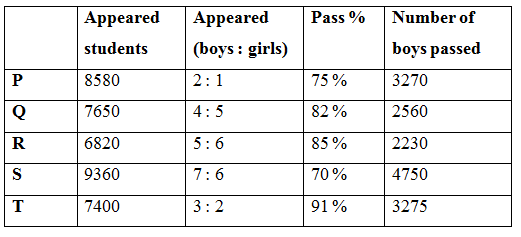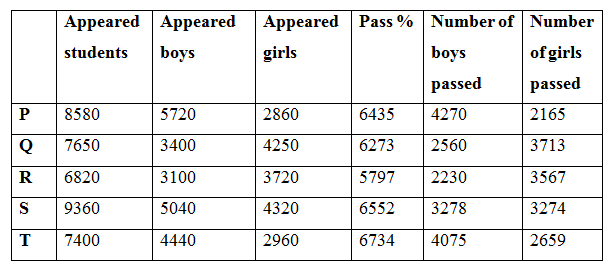# NIACL AO Mains– Quantitative Aptitude Questions Day- 16

Dear Readers, Bank Exam Race for the Year 2019 is already started, To enrich your preparation here we have providing new series of Practice Questions on Quantitative Aptitude – Section. Candidates those who are preparing for NIACL AO Mains 2019 Exams can practice these questions daily and make your preparation effective.

[WpProQuiz 5224]

Directions (Q. 1 – 5) In the following questions, two equations I and II are given. You have to solve both the equations and give answer as,

a) If x > y

b) If x ≥ y

c) If x < y

d) If x ≤ y

e) If x = y or the relation cannot be established

1) I) x2 + 19x – 92 = 0

II) 2y2 – 15y – 38 = 0

2) I) 3x2 + 23x + 44 = 0

II) 4y2 – 6y – 28 = 0

3) I) 6x – 7y + 2 = 3x – 2y

II) 2x – 3y = 0

4) I) x = ∛19683 + 52 ÷ 4

II) y = √2401 – 32

5) I) 2x2 – 25x + 77 = 0

II) 3y2 – 23y + 42 = 0

Directions (Q. 6 – 10) Study the following information carefully and answer the given questions:

The following table shows the total number of students appeared for the examination from different cities and the ratio of appeared boys to girls among them and the percentage of students passed in the examination and number of boys passed in the examination.6) Find the average number of girls appeared for the examination from all the given cities together?

a) 3156

b) 3480

c) 3622

d) 3378

e) None of these

7) The total number of girls passed in the city Q and R together is approximately what percentage of total number of girls passed in the city S and T together?

a) 156 %

b) 112 %

c) 145 %

d) 123 %

e) 137 %

8) Find the difference between the total number of girls appeared for the examination to that of total number of boys appeared for the examination from all the given cities together?

a) 4520

b) 3590

c) 2470

d) 5350

1. e) None of these

9) Find the ratio between the total number of girls passed from the city P and S together to that of total number of boys passed from the same city?

a) 1813 : 2516

b) 1134 : 1785

c) 1542 : 2379

d) 2156 : 2893

e) None of these

10) Total number of students appeared for the examination from city R and S together is approximately what percentage more/less than the total number of girls appeared for the examination from all the given cities together?

a) 39 % more

b) 11 % more

c) 11 % less

d) 39 % less

e) 8 % more

Direction (1-5)  :

I) x2 + 19x – 92 = 0

(x + 23) (x – 4) = 0

X = -23, 4

II) 2y2 – 15y – 38 = 0

2y2 + 4y – 19y – 38 = 0

2y (y + 2) -19 (y + 2) = 0

(2y – 19) (y + 2) = 0

Y = 19/2, -2 = 9.5, -2

Can’t be determined

I) 3x2 + 23x + 44 = 0

3x2 + 12x + 11x + 44 = 0

3x (x + 4) + 11 (x + 4) = 0

(3x + 11) (x + 4) = 0

X = -11/3, -4 = -3.66, -4

II) 4y2 – 6y – 28 = 0

4y2 + 8y – 14y – 28 = 0

4y (y + 2) -14 (y + 2) = 0

(4y – 14) (y + 2) = 0

Y = 14/4, -2 = 3.5, -2

X < y

I) 6x – 7y + 2 = 3x – 2y

3x – 5y = -2 —> (1)

II) 2x – 3y = 0 —> (2)

By solving the equation (1) and (2), we get,

X = 6, y = 4

X > y

I) x = ∛19683 + 52 ÷ 4

X = 27 + (52/4)

X = 27 + 13 = 40

II) y = √2401 – 32

Y = 49 – 9 = 40

X = y

I) 2x2 – 25x + 77 = 0

2x2 – 11x – 14x + 77 = 0

X (2x – 11) – 7 (2x – 11) = 0

(x – 7) (2x – 11) = 0

X = 7, 11/2 = 7, 5.5

II) 3y2 – 23y + 42 = 0

3y2 – 9y – 14y + 42 = 0

3y (y – 3) -14(y – 3) = 0

(3y – 14) (y – 3) = 0

Y = 14/3, 3 = 4.66, 3

X > y

Direction (6-10) :The total number of girls appeared for the examination from all the given cities together

= > 8580*(1/3) + 7650*(5/9) + 6820*(6/11) + 9360*(6/13) + 7400*(2/5)

= > 2860 + 4250 + 3720 + 4320 + 2960

= > 18110

Required average = 18110/5 = 3622

The total number of girls passed in the city Q and R together

= > 3713 + 3567 = 7280

The total number of girls passed in the city S and T together

= > 3274 + 2659 = 5933

Required % = (7280/5933)*100 = 122.70 % = 123 %

The total number of girls appeared for the examination from all the given cities together

= > 8580*(1/3) + 7650*(5/9) + 6820*(6/11) + 9360*(6/13) + 7400*(2/5)

= > 2860 + 4250 + 3720 + 4320 + 2960

= > 18110

The total number of boys appeared for the examination from all the given cities together

= > 8580*(2/3) + 7650*(4/9) + 6820*(5/11) + 9360*(7/13) + 7400*(3/5)

= > 5720 + 3400 + 3100 + 5040 + 4440

= > 21700

Required difference = 21700 – 18110 = 3590

The total number of girls passed from the city P and S together

= > 2165 + 3274 = 5439

The total number of boys passed from the city P and S together

= > 4270 + 3278 = 7548

Required ratio = 5439 : 7548 = 1813 : 2516

Total number of students appeared for the examination from city R and S together

= > 6820 + 9360 = 16180

Total number of girls appeared for the examination from all the given cities together

= > 2860 + 4250 + 3720 + 4320 + 2960 = 18110

Required % = [(18110 – 16180)/18110]*100 = 10.65 % = 11 % less